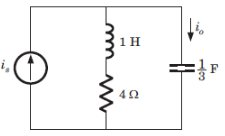Courses

# Test: Circuit Analysis In S Domain

## 10 Questions MCQ Test Electronic Devices | Test: Circuit Analysis In S Domain

Description
This mock test of Test: Circuit Analysis In S Domain for Electrical Engineering (EE) helps you for every Electrical Engineering (EE) entrance exam. This contains 10 Multiple Choice Questions for Electrical Engineering (EE) Test: Circuit Analysis In S Domain (mcq) to study with solutions a complete question bank. The solved questions answers in this Test: Circuit Analysis In S Domain quiz give you a good mix of easy questions and tough questions. Electrical Engineering (EE) students definitely take this Test: Circuit Analysis In S Domain exercise for a better result in the exam. You can find other Test: Circuit Analysis In S Domain extra questions, long questions & short questions for Electrical Engineering (EE) on EduRev as well by searching above.
QUESTION: 1

### The network function (s2 + 4s)/(s + 1)(s + 2)(s + 3) represents

Solution:

Poles and zero does not interlace on negative real axis so it is not a immittance function.

QUESTION: 2

### The network function (3s2 + 8s)/(s + 1)(s + 3) represents

Solution:

The singularity nearest to origin is a zero. So it may be RL impedance or RC admittance function. Because of (D) option it is required to check that it is a valid RC admittance function. The poles and zeros interlace along the negative real axis. The residue of Yrc(s)/s is positive.

QUESTION: 3

### The network function (s + 1)(s + 4)/s(s + 2)(s + 5) represents

Solution:

The singularity nearest to origin is a pole. So it may be RC impedance or RL admittance function.

QUESTION: 4

The network function s^2 + 10s + 24/s2 + 8s + 15 represents

Solution:

The singularity is near to origin is pole. So it may be RC impedance or RL admittance function.

QUESTION: 5

A valid immittance function is

Solution:

a) pole lie on positive real axis
b) poles and zero does not interlace on axis.
c) poles and zero does not interlace on axis.
d) is a valid immittance function.

QUESTION: 6

The network function (s2 + 8s +15)/(s2 + 6s + 8) is

Solution:

The singularity nearest to origin is a pole. So it may be a RL admittance or RC impedance function.

QUESTION: 7

The voltage response of a network to a unit step input is Vo(s) = 10/s(s2 + 8s + 16). The response is

Solution:

The characteristic equation has real and repeated roots (-4, -4). Hence it is critically damped.

QUESTION: 8

The current response of a network to a unit step input is Io(s) = 10(s + 2)/s(s2 + 11s + 30). The response is

Solution:

The roots are real and unequal (-6, -5) for the characteristic equation. Hence it is over damped.

QUESTION: 9Q. The ratio of the transfer function Io/Is is

Solution:

Io/Is = (s + 4)/ (s + 4 + 3/s) = s(s + 4)/(s + 1)(s + 3).

QUESTION: 10Q. The response is

Solution:

The characteristic roots are real and unequal (-1, -3), therefore it is over damped.